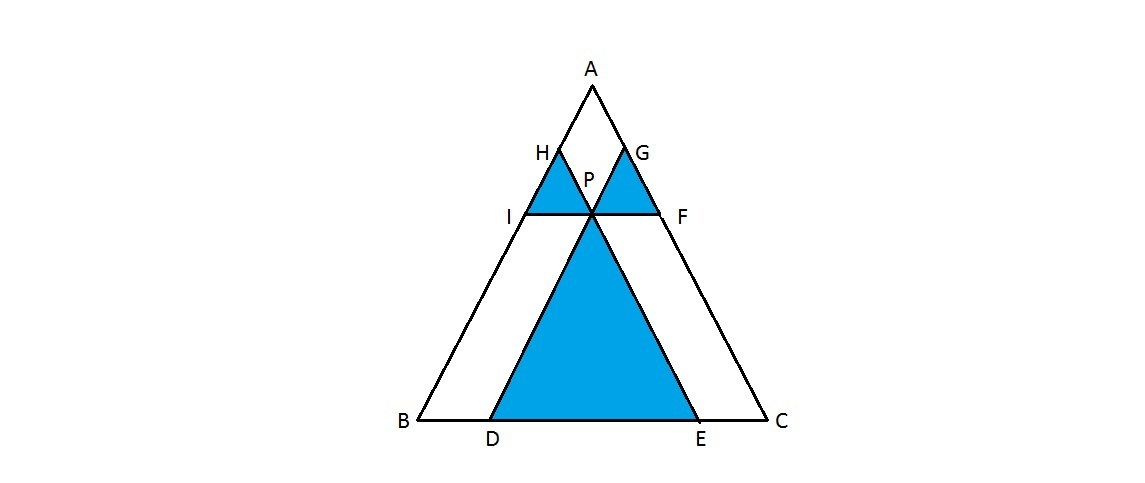# Insufficient Information On Areas?

Geometry Level 4If P is a point inside the triangle ABC, and line segments DPG, HPE, FPI are drawn parallel to sides AB, AC and BC respectively through the point P. If area of triangles PDE, PFG and PHI are 36, 9 and 4 respectively, find the area of triangle ABC.

×